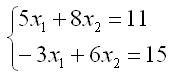favorite We need a little bit of your help to keep things running, click on this banner to learn more
Problems

# Cramer's Formula

Solve the system of two linear equations with two variables using the Kramer's rule.

The system of equations given in the example has the form:It is known that system has unique solution.

#### Input

The first line contains the coefficients of the first equation, and the second line contains the coefficients of the second equation. All numbers are separated with one space and do not exceed 100 by the absolute value.

#### Output

Print the first root in the first line and the second root in the second line with accuracy 0.001.

Time limit 1 second
Memory limit 128 MiB
Input example #1
5 8 11
-3 6 15

Output example #1
-1.000
2.000